# Consistent estimator

(diff) ← Older revision | Latest revision (diff) | Newer revision → (diff)

An abbreviated form of the term "consistent sequence of estimators" , applied to a sequence of statistical estimators converging to a value being evaluated.

In probability theory, there are several different notions of the concept of convergence, of which the most important for the theory of statistical estimation are convergence in probability and convergence with probability 1. If a sequence of statistical estimators converges in probability to the value being evaluated, then one says that this sequence is "weakly consistent estimatorweakly consistent" or simply "consistent" , and one reserves the term "strongly consistent estimatorstrong consistency" for a sequence of estimators that converges with probability 1 to the value being evaluated.

Example 1)

Letbe independent random variables with the same normal distribution. Then the statistics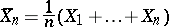and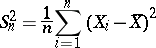are consistent estimators for the parametersand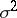, respectively.

Example 2) Letbe independent random variables subject to the same probability law, the distribution function of which is. In this case, the empirical distribution function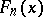constructed from an initial sampleis a consistent estimator of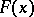.

Example 3) Letbe independent random variables subject to the same Cauchy law, whose probability density is. For any natural number, the statisticis subject to the initial Cauchy law, hence the sequence of estimators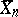does not converge in probability to, that is, in the given example the sequenceis not consistent. A consistent estimator forhere is the sample median.

A consistent estimator has the following property: Ifis a continuous function and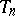is a consistent estimator of a parameter, then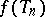is a consistent estimator for. The most common method for obtaining statistical point estimators is the maximum-likelihood method, which gives a consistent estimator. It must be noted that a consistent estimatorof a parameteris not unique, since any estimator of the formis also consistent, where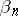is a sequence of random variables converging in probability to zero. This fact reduces the value of the concept of a consistent estimator.

How to Cite This Entry:
Consistent estimator. Encyclopedia of Mathematics. URL: http://encyclopediaofmath.org/index.php?title=Consistent_estimator&oldid=14325
This article was adapted from an original article by M.S. Nikulin (originator), which appeared in Encyclopedia of Mathematics - ISBN 1402006098. See original article